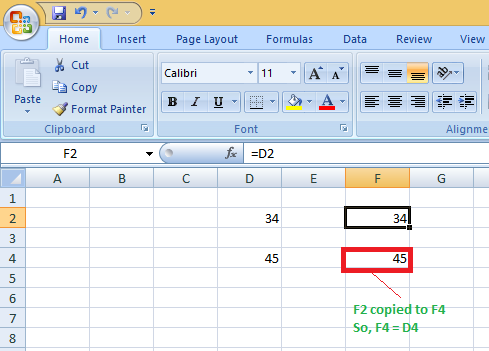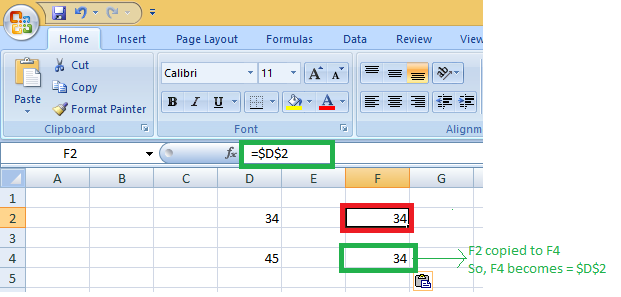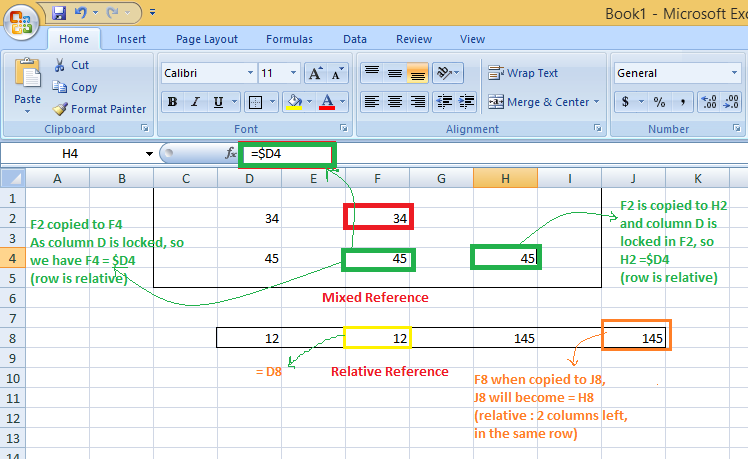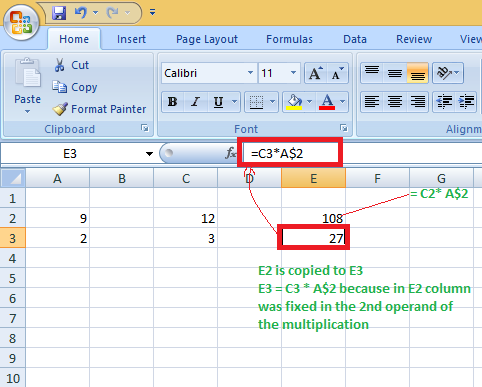# Mixed Reference in Excel

A cell reference or a cell address is a way to define a cell on a worksheet, it is formed by combining a column letter and a row number. To refer any if the cell on a worksheet (in any formula/ to copy the cell) we use cell reference.

Example: To refer to the cell present in row 2 & column D, cell reference is: D2, where D is column letter & 2 is the row number.

### Relative Cell Reference :

By default, every cell is a relative reference. Example: If you refer to cell D2 in cell F2, you refer to a cell that is 2 columns to the left (F-D = 3) in the same row number 2.

The relative relation for that location will be updated automatically when it is copied to other positions in a worksheet. (Because a relative cell reference defines an offset to another cell – say, 2 columns left within the same row in the example mentioned above).

If you copy F2 to F4, then F4 = D4 (as F2 = D2, i.e., 2 columns left in the same row), So D4 is two columns left to F4 in the same row 4.### Absolute Cell Reference :

There are times when the cell reference must stay the same when copying or using AutoFill. Using dollar signs, a column and/or row reference can be kept constant. So, to get an absolute reference from a relative, we can use the dollar sign (\$) characters.

We use an absolute reference to refer to an actual fixed location on a worksheet whenever copying is done. The reference here is locked such that rows and columns do not shift when copied.

Dollar (\$) before row fix the row & before the column fixes the column.

Example: Fixing/Locking both – row & column – Say if we want to lock  row 2 & column D, we will use \$D\$2 as:

F2 = \$D\$2, when copied to F4, F4 also becomes = \$D\$2.### Excel Mixed Cell Reference :

A mixed cell reference is a mixture of absolute & relative reference, i.e., an absolute column and relative row, or an absolute row and relative column. To get an absolute column or absolute row, you can put the \$ before the column letter or before the row number.

Example: \$D2 is relative for row 2 but absolute for column D, and D\$2 is absolute for row 2 but relative for column D.

Here Dollar (\$) before row number fixes/locks the row & before the column name fixes/locks the column.

Example 1: When we fix the only row: If we have F2 =\$D2 then :

We used \$ before the column number, so we are locking the only column here.When F2 is copied to H4, then H4 = \$D4 because the column is the same here, i.e., column letter D But the row is relative (i.e. the same row in which copying is done).

Example 2: When we fix /lock the only row : If we have E2 = C2*A\$2 then :

When E2 is copied to E3, E3 = C3*A\$2 (not C3*A3) because the row (number 2) has been locked already.I hope you now know the relative and absolute cell references, how to use \$ sign and how to use mixed cell references.

Whether you're preparing for your first job interview or aiming to upskill in this ever-evolving tech landscape, GeeksforGeeks Courses are your key to success. We provide top-quality content at affordable prices, all geared towards accelerating your growth in a time-bound manner. Join the millions we've already empowered, and we're here to do the same for you. Don't miss out - check it out now!